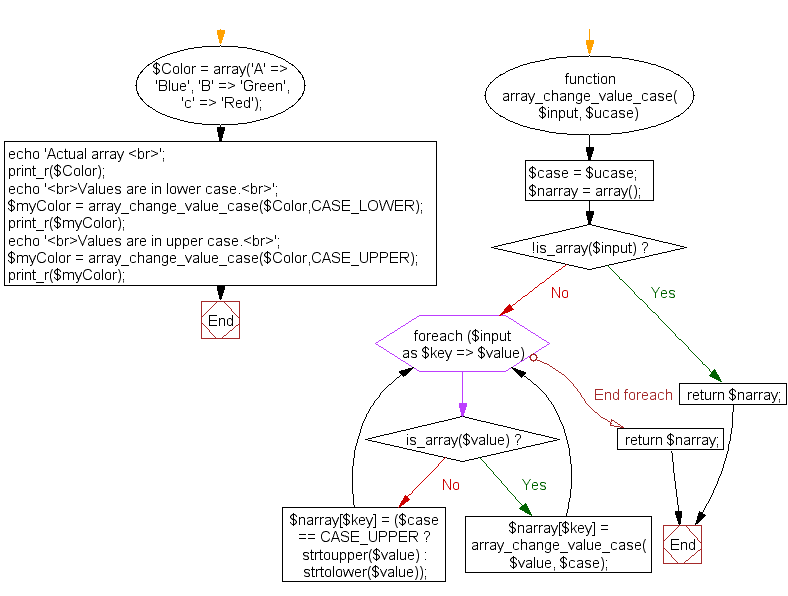﻿ PHP Array Exercise: Change the array values to upper or lower case - w3resource# PHP Array Exercises : Change the array values to upper or lower case

## PHP Array: Exercise-12 with Solution

Write a PHP function to change the following array's all values to upper or lower case.

Sample arrays :
\$Color = array('A' => 'Blue', 'B' => 'Green', 'c' => 'Red');

Sample Solution:

PHP Code:

``````<?php
function array_change_value_case(\$input, \$ucase)
{
\$case = \$ucase;
\$narray = array();
if (!is_array(\$input))
{
return \$narray;
}
foreach (\$input as \$key => \$value)
{
if (is_array(\$value))
{
\$narray[\$key] = array_change_value_case(\$value, \$case);
continue;
}
\$narray[\$key] = (\$case == CASE_UPPER ? strtoupper(\$value) : strtolower(\$value));
}
return \$narray;
}
\$Color = array('A' => 'Blue', 'B' => 'Green', 'c' => 'Red');
echo 'Actual array ';
print_r(\$Color);
echo 'Values are in lower case.';
\$myColor = array_change_value_case(\$Color,CASE_LOWER);
print_r(\$myColor);
echo 'Values are in upper case.';
\$myColor = array_change_value_case(\$Color,CASE_UPPER);
print_r(\$myColor);
?>
```
```

Sample Output:

```Actual array Array
(
[A] => Blue
[B] => Green
[c] => Red
)
Values are in lower case.Array
(
[A] => blue
[B] => green
[c] => red
)
Values are in upper case.Array
(
[A] => BLUE
[B] => GREEN
[c] => RED
)
```

Flowchart:PHP Code Editor:

What is the difficulty level of this exercise?

Test your Programming skills with w3resource's quiz.

﻿

## PHP: Tips of the Day

Concatenation Operators: You can use concatenation to join strings "end to end" while outputting them (with echo or print).

You can concatenate variables using a . (period/dot).

Example:

```<?php
// String variable

\$name = 'Jhon';

// Concatenate multiple strings (3 in this example) into one and echo it once done.

echo '<p>Hello ' . \$name . ', Nice to meet you.</p>';

// Concatenation Operators
?>
```

Output:

```<p>Hello Jhon, Nice to meet you.</p>
```

Similar to concatenation, echo (when used without parentheses) can be used to combine strings and variables together (along with other arbitrary expressions) using a comma (,).

```<?php
\$itemCount = 1;
echo 'You have learn ', \$itemCount, ' Tips', \$itemCount === 1 ? '' : 's';
?>
```

Output:

```You have learn 1 Tips
```

String concatenation vs passing multiple arguments to echo

Passing multiple arguments to the echo command is more advantageous than string concatenation in some circumstances. The arguments are written to the output in the same order as they are passed in.

```echo "The total is: ", \$x + \$y;
```

The problem with the concatenation is that the period . takes precedence in the expression. If concatenated, the above expression needs extra parentheses for the correct behavior. The precedence of the period affects ternary operators too.

```echo "The total is: " . (\$x + \$y);
```